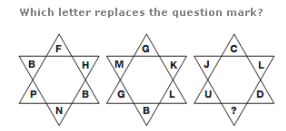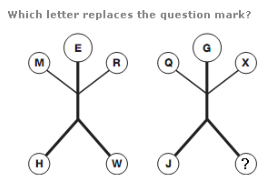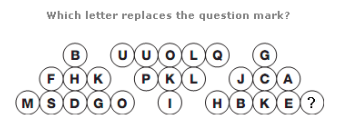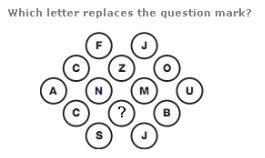21.A. T B. B C. H D. D

Explanation: :

Start at the top and move down, taking single letters or pairs of letters that appear on the same horizontal line.

The sum of the numerical values of the letters in each line increases by 2 as you go down, from 18 to 26.

22.A. U B. C C. M D. F

Explanation: :

In each star shape, the sum of the numerical values of the letters bounded by one triangle is equal to that of the other triangle.

23.A. E B. R C. T D. Y

Explanation: :

In each stick-man diagram, start on the left foot and move around the other limbs in a clockwise direction.

The letters advance through the alphabet in steps given by the numerical value of the letter on the head of the diagram.

24.A. W B. B C. E D. R

Explanation: :

Add together the numerical values of the letters in corresponding positions on the left and right hand triangles, and put the letter with this value in corresponding segments of the centre triangle.

25.A. A B. Z C. K D. P